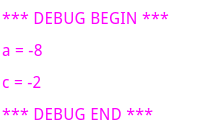Index

Working with MUMIE as author

• Initial steps:
• Articles:
• Problems:
• Programming with Python
• New Visualizations with JSXGraph
• Old Visualizations:
• Media Documents:

Working with MUMIE as teacher

Using MUMIE via plugin in local LMS

FAQ for examination lecturers

Overview

Go back to the old wiki

We revise and update this wiki. We apologize for the inconvenience this may cause you.

# Variables

Questions can become dynamic and personalised through the use of variables and functions.
These must be defined in a variables environment

The environment \begin{variables} ... \end{variables} can be set for the whole group of questions, but can also be set individually for each question, or both. The position of the command determines for which questions the variables will be valid.

1234567891011121314151617\begin{problem}     \begin{variables} % set for all questions      ...    \end{variables}     \begin{question}      \begin{variables} % set only for this questions        ...      \end{variables}       \begin{answer}        ...      \end{answer}               \end{question}         \end{problem} 

# Example

1234567891011\begin{question}   \begin{variables}       \number{a}{12}       \number{b}{4}       \number{c}{10}       \number{d}{5}       \function{f}{a/b}       \function{g}{c/d}   \end{variables}   ...\end{question} 

Will result in variables

• $$a=12$$,
• $$b=4$$,
• $$c=10$$,
• $$d=5$$ and
• functions $$f=\frac{12}{4}$$ and $$g=\frac{10}{5}$$

# Names of variables

The names of your variables are restricted to some constraints:

• It must only contain the standard letters (a-z and A-Z), digits (0-9), and underscore (_ ),
• It has to begin with a standard letter (a-z or A-Z),
• It must not be equal to a predefined constant or function (see expression syntax)

It is not excluded that other names may also work, but it is highly recommended to stick to the constraints given above.

Variable names are case sensitive, e.g.

1234\begin{variables}       \number{a}{12}       \function{A}{a+2}\end{variables} 

results in two variables, one named a with value 12 and one named A with value 14.

# Commands

Command Description Details
\number A fixed number variable, this can be any kind of number. The field command determines how the number will be interpreted.
\function A function definition, the definition may include variables and other functions.
When an included variable is not defined, it will be a free variable.
Again, the field command determines how the numbers within the function will be interpreted.
function
\derivative Creates a new function representing the derivative of the expression/variable that was passed into it. derivative
\substitute Creates a new function where the free variable of a function is replaced by the value of another function variable. substitute
\matrix A matrix displayed with square brackets input.matrix questions
\pmatrix A matrix displayed with round brackets input.matrix questions
\string A string definition as needed for input.text questions.
When combined with switch/case-environments, it can be used to show different text depending on random variables.
input.text questions
\randint A random integer number, including zero (same as randint[z])
\randint[Z] A random integer number, excluding zero Random numbers
\randdouble A random double number, including zero Random numbers
\randrat A random rational number, including zero Random numbers
\drawFromSet Randomly draw a number (or string) from a set Random numbers
\randadjustIf Random variables can be adjusted to satisfy a specific constraint. randadjustIf

# Using Variables in text

All variables can be used in texts (e.g. question task, answer text) by using the tex command \var with the name of the variable as argument:

12345678910111213\begin{question}   \begin{variables}    \function{f}{a/3}  \end{variables}   \text{Determine the decimal expansion of $\var{f}$ correct to three decimal places.}   \begin{choice}    \text{$\var{solution1}$}  \end{choice} \end{question} 

# Random numbers

Random numbers can be used instead of fixed numbers to personalize problem data.

Example

123456789\begin{question}   \begin{variables}      \randint[Z]{x1}{-10}{10}  %\randint[Z]{name}{min}{max}      \randdouble{x2}{0}{1}      \randrat{name}{minNumerator}{maxNumerator}{minDenominator}{maxDenominator}  \end{variables} \end{question} 

\drawFromSet Draw randomly a number (or string) from a set

• parameters \drawFromSet[options]{name}{set}
• numbers in the set can be integer, rational or double numbers
• separator in the set can be any (non white space) character, default separator is the comma
• type is either number or string, default type is number
• multiple options are separated by a space (see example below)
12345678910\begin{question}   \begin{variables}      \drawFromSet{a}{1,2,3,4,5,6}      \drawFromSet{b}{1/2,5,1.3,9/11,42}      \drawFromSet[separator=;]{c}{1;2.1;3.5;9}      \drawFromSet[separator=; type=string]{d}{hello;world}  \end{variables} \end{question} 

If you randomize variables, it could happen that the random values do not satisfy some conditions the author wishes to
be fulfilled in the question, or that the problem is not correctly posed for some combinations of the random values.
So they have to be adjusted. Therefore, you can use the following syntax to achieve this:

\randadjustIf{ _list_of_variables_ }{ _avoidance_relation_ }

A detailed explanation is given in RandadjustIf.

# Switch/Case statements

There is a switch/case statement to make sure your variables satisfy specific constraints.

12345678910111213141516\begin{switch}  \begin{case}{condition1}    ... variable definitions ...  \end{case}   \begin{case}{condition2}    ... variable definitions ...  \end{case}   ...   \begin{default}    ... variable definitions ...  \end{default} \end{switch} 

Every variable in a switch environment must have a default definition, but not a definition for every single case environment
(see example below). There is no limitation on how many case environments you can use inside a switch environment. The default environment though is obligatory!

You can use switch/case statements globally and on question level.

Note: Never use switch/case statements in combination with \randadjustIf. It is meant as an alternative.

An example:

1234567891011121314151617181920212223242526272829303132\begin{variables}\randint{a}{1}{5}  \function{f0}{10*a}   \begin{switch}    \begin{case}{a>3}      \number{c}{3}      \randrat{d}{1}{2}{3}{7}      \drawFromSet{m}{1,2,3}      \function{f}{2*a}      \derivative{g}{3x^2+sqrt(x)}{x}      \substitute{h}{sqrt(y)}{y}{g}      \string{s}{case 1}    \end{case}     \begin{case}{a=3}      \number{c}{5}    \end{case}     \begin{default}      \randint{c}{1}{10}      \randrat{d}{-2}{-1}{3}{7}      \drawFromSet{m}{-1,-2,-3}      \function{f}{-2*a}      \derivative{g}{-3x^2+sqrt(x)}{x}      \substitute{h}{sqrt(y)}{y}{g}      \string{s}{default case}    \end{default}   \end{switch} \end{variables} 

# Variable pools

There is also the option to define several sets of
values inside a so called variable pool.

• A pool consists of several variables environments holding each the elements for a single set of variables.
12345678910111213141516171819\begin{question}   \begin{pool}    \begin{variables}      \randint{a}{1}{3}      \drawFromSet{b}{1,2,3}      \randadjustIf{a,b}{a = b}      \function[calculate]{f}{2*a+b}    \end{variables}         \begin{variables}      \randint{a}{-3}{-1}      \drawFromSet{b}{-1,-2,-3}      \randadjustIf{a,b}{a = b}      \function[calculate]{f}{2*a-b}    \end{variables}      \end{pool} \end{question} 
• A single set will be chosen randomly for every homework problem. The following example shows a pool with two sets of variables:
1234567891011121314151617\begin{question}   \begin{pool}       \begin{variables}          \number{a}{7}          \number{b}{3}      \end{variables}       \begin{variables}          \number{a}{5}          \number{b}{6}      \end{variables}   \end{pool} \end{question} 
Variable pools can only be used inside a question. There are no global variable pools at the moment.

# Variable Debug Output

For developing/debugging purposes in WebMiau it's sometimes helpful to look at the variable values, especially the ones that are not displayed in the problem, but essential for the automatic correction. You can use \debug[<comma separated list of variable names>] within a question environment to display the random variable values in the preview of a problem. \debug without the optional argument will display the random values of all variables.

Don't forget to remove the \debug command from the TeX source before publishing the problem to a server or to the problem pool.

Example

1234567891011\begin{question}   \begin{variables}      \randint[Z]{a}{-10}{10}      \randdouble{b}{0}{1}      \drawFromSet{c}{-1,-2,-3}  \end{variables}   \debug[a,c] \end{question}